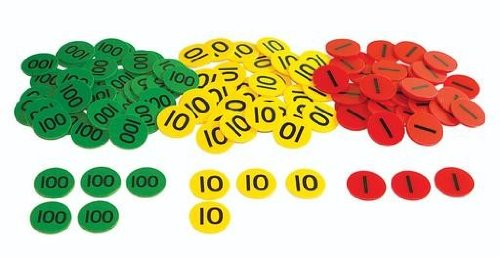# Maths problems of the week

### Problem 1

Milly saysI know that  4 x 20 = 80  .  I also know that 4 x 3 = 12

Explain how she can use those facts to work out 4 x 23

### Problem 2

Calculate 3  x 16     using the grid method and counters that we have learnt last week

use a gridand place value counters## 6 thoughts on “Maths problems of the week”

1.Neel jadav

So if know 4×20=80 and if you add 4×3=12and if add them together =92 so that’s how you know 4×23=92.

Like

2.Samir Parvez

Problem 1
1. You partition 23 it is 20 and 3.
2. Write 20 x 3=60 and write 4 x 3=12.
3. Add 60 and 12 and it =72

It will help you because I know that 20 x 3=60 and 4 x 3=12

Like

3.Samir Parvez

Problem 2
X 10 6
3 30 18

30 + 18 = 48
16 x3=48

Like

4.Yusof

What milly has done is that she first knows
4times 20 is 80. And then it was 4 times 3
is 12 but the important question was 4 times 23.what she did she partitioned
The 2 into 20.and then she partitioned 3 . The real answer was 93 for the question 4

Like

5.Yusof

If I know that 4times20=80 then I would do 4 times 3=12 but our question is what is 4 times 23• 4 times 23=92. That fact was you had to know is 4 times 20 is and you had to know is 4 times 3 is

Like

6.Yusof

If I know that 4 times 20 =20 then I also have to know what 4 times 3=12.But our real question was 4 times 23=92.But our real facets you had to know is 4 times 20 and 4 times 3 and the final clue is 4 times 23

Like